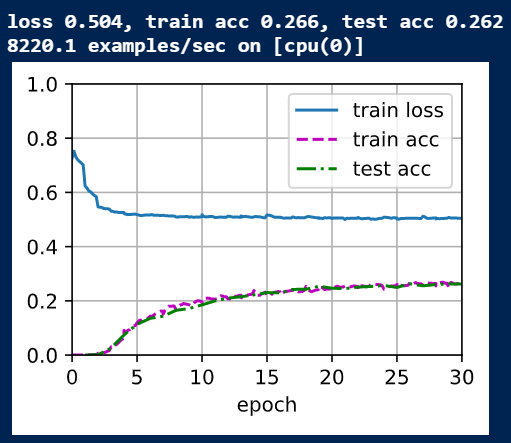# Factorization Machineswhere does the field_dim come from?

I think there might be a bug in the evaluation step of the model. To measure accuracy, the current d2l.accuracy function casts the predictions to the same type as y then compares how many are the same. Issue is although y is a float it is binary 0 or 1, and we are comparing probabilities to binary, so unless the model perfectly says something is a 0 or 1 all other values are treated as misclassification. I did a simple comparison of the original accuracy calc `(d2l.astype(net(X),y.dtype) == y).sum()` vs `(round(net(X)) == y).sum()` and got wildly different results. Test was done on a single batch using `X,y=next(iter(test_iter))`. The round method puts a threshold @ 50%, and in one batch using this i got 1896 labels classified correctly vs the original method where only 536 were shows as classified correctly.

What’s the difference between MF and FM?